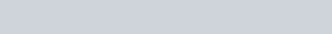A boat is moving due east in a region where the earth’s magnetic field is
Question:

A boat is moving due east in a region where the earth’s magnetic field is $5.0 \times 10^{-5} \mathrm{NA}^{-1} \mathrm{~m}^{-1}$ due north and horizontal. The boat carries a vertical aerial $2 \mathrm{~m}$ long. If the speed of the boat is $1.50 \mathrm{~ms}^{-1}$, the magnitude of the indueced emf in the wire of aerial is :-

1.  $0.50 \mathrm{mV}$

2.  $0.15 \mathrm{mV}$

3.  $1 \mathrm{mV}$

4. $0.75 \mathrm{mV}$

Correct Option: , 2

Solution: